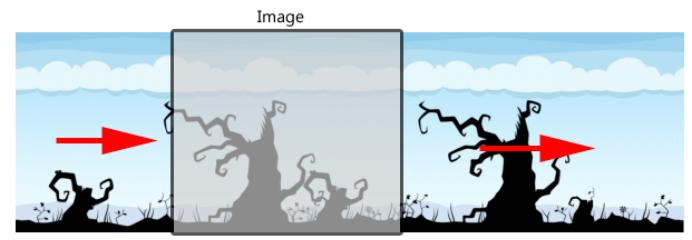Shader "Lpy/ImageRoll"
{
Properties
{
_MainTex ("Main Tex", 2D) = "white" {}

_Width ("Width", float) = 0.5
_Distance ("Distance", float) = 0
}

{
Tags {"Queue"="Transparent" "IgnoreProjector"="True" "RenderType"="Transparent"}

Pass
{
Tags { "LightMode"="ForwardBase" }
ZTest off
ZWrite Off
Blend SrcAlpha OneMinusSrcAlpha

CGPROGRAM
#pragma vertex vert
#pragma fragment frag
#include "UnityCG.cginc"

sampler2D _MainTex;
float _Width;
float _Distance;

struct a2v
{
float4 vertex : POSITION;
float2 texcoord : TEXCOORD0;
};

struct v2f
{
float4 pos : SV_POSITION;
float2 uv : TEXCOORD0;
};

v2f vert (a2v v)
{
v2f o;
o.pos = mul(UNITY_MATRIX_MVP, v.vertex);

o.uv.x = v.texcoord.x;
o.uv.y = v.texcoord.y;
return o;
}

fixed4 frag (v2f i) : SV_Target
{
i.uv.x = frac(i.uv.x*_Width + _Distance);
fixed4 c = tex2D(_MainTex, i.uv);
return c;
}
ENDCG
}
}
FallBack "Transparent/VertexLit"
}

## 3、代码中引用

using UnityEngine;
using System.Collections;
using UnityEngine.UI;

public class ImageRoll : MonoBehaviour {

public Image image;
private Material mtl;
float distance;
// Use this for initialization
void Start () {
mtl = image.material;
}

// Update is called once per frame
void Update () {
distance += 0.005f;
mtl.SetFloat("_Distance", distance);
}
}